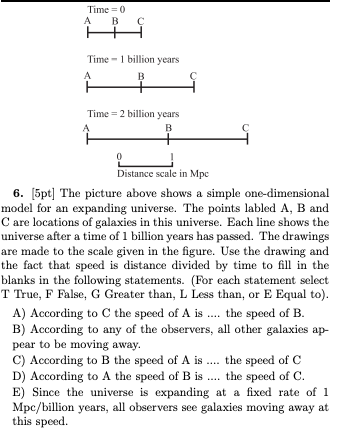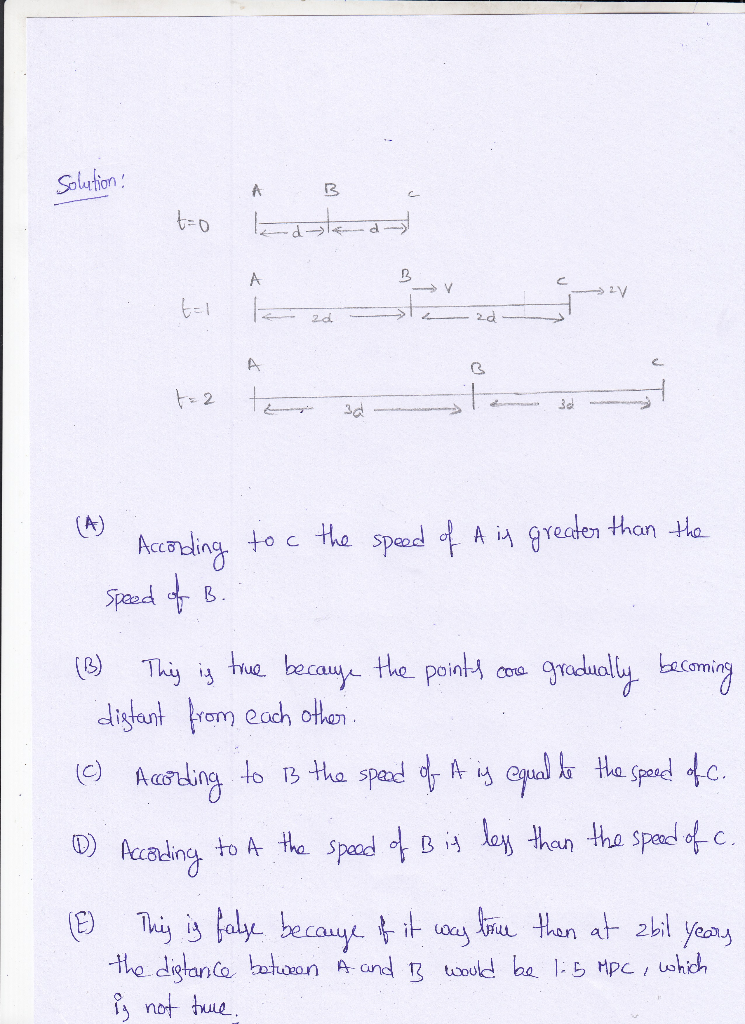Homework Help Question & Answers

# Time 0 A B C Time-1 billion years Time 2 billion years Distance scale in Mpc 6. [5pt] The picture above shows a simple one-dimensional model for an expanding universe. The points labled A, B and C ar...Time 0 A B C Time-1 billion years Time 2 billion years Distance scale in Mpc 6. [5pt] The picture above shows a simple one-dimensional model for an expanding universe. The points labled A, B and C are locations of galaxies in this universe. Each line shows the universe after a time of 1 billion years has passed. The drawings are made to the scale given in the figure. Use the drawing and the fact that speed is distance divided by time to fill in the blanks in the following statements. (For each statement select T True, F False, G Greater than, L Less than, or E Equal to). A) According to C the speed of A is the speed of B. B) According to any of the observers, all other galaxies ap pear to be moving away. C) According to B the speed of A is D) According to A the speed of B is. the speed of C. the speed of C E) Since the universe is expanding at a fixed rate of 1 Mpc/billion years, all observers see galaxies moving away at this speed##### Add Answer of: Time 0 A B C Time-1 billion years Time 2 billion years Distance scale in Mpc 6. [5pt] The picture above shows a simple one-dimensional model for an expanding universe. The points labled A, B and C ar...
More Homework Help Questions Additional questions in this topic.

• #### Suppose that S(savings) = \$4 billion when Real GDP = \$200 billion & S(savings) = \$104 billion when Real GDP = \$600 billion

Need Online Homework Help?

Get FREE EXPERT Answers
WITHIN MINUTES
Related Questions